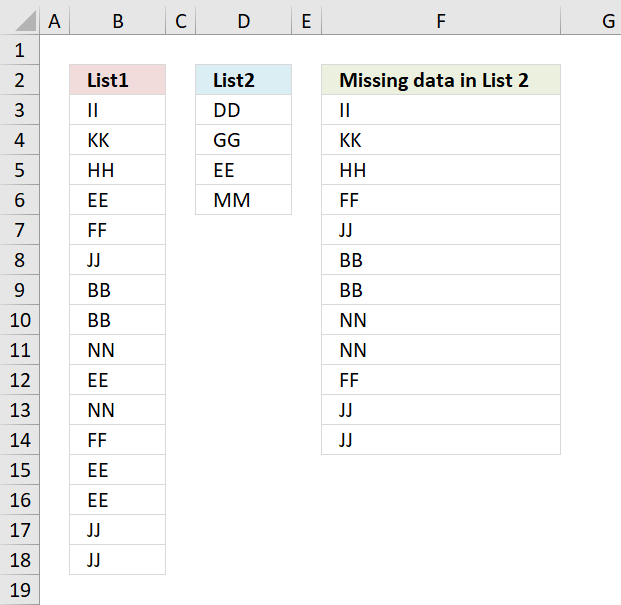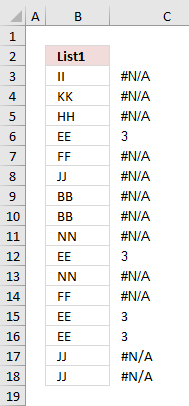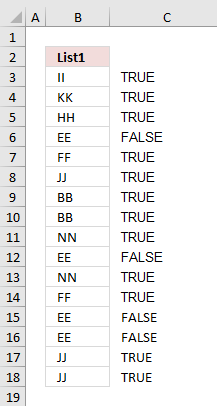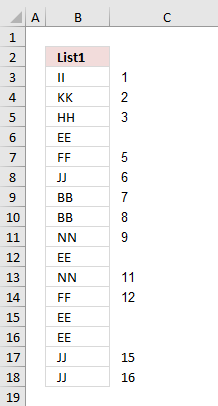Author: Oscar Cronquist Article last updated on August 10, 2018Question: How to filter out data from List 1 that is missing in list 2?

Answer: This formula is useful when comparing two lists to find out what cell values are missing.For instance, inventory comparison.

=INDEX(\$B\$3:\$B\$18, SMALL(IF(ISERROR(MATCH(\$B\$3:\$B\$18, \$D\$3:\$D\$6, 0)), (ROW(\$B\$3:\$B\$18)-MIN(ROW(\$B\$3:\$B\$18))+1), ""), ROWS(\$A\$1:A1)))

How to create an array formula

1. Select cell c2
2. Click in formula bar
3. Paste above array formula to formula bar4. Press and hold Ctrl + Shift
5. Press Enter
6. Release all keys

You know you have entered an array formula when the formula in the formula bar is surrounded by curly brackets {=array_formula}

Explaining formula

Step 1 - Compare values between A2:A17 and B2:B5The MATCH function looks for a specific value in a cell range or array and returns it's position in that cell range or array.

If the value does not exist in the cell range or array the MATCH function returns #N/A (error value).

MATCH(\$A\$2:\$A\$17, \$B\$2:\$B\$5, 0)

checks if there are any matches. If there are none, an error will occur.

{#N/A,#N/A,#N/A, 3,#N/A,#N/A,#N/A,#N/A,#N/A, 3,#N/A,#N/A, 3, 3,#N/A,#N/A,}

The image to the right shows the array and the corresponding value in column B. It is quite obvious already now which values are missing in the List 2 and which ones that exist.

Step 2 - Identify error values in arrayIf a value in array is an error the ISERROR function returns TRUE else FALSE.

ISERROR(MATCH(\$A\$2:\$A\$17, \$B\$2:\$B\$5, 0))

becomes

ISERROR({#N/A,#N/A,#N/A, 3,#N/A,#N/A,#N/A,#N/A,#N/A, 3,#N/A,#N/A, 3, 3,#N/A,#N/A,})

and returns

{TRUE, TRUE, TRUE, TRUE, FALSE, TRUE, TRUE, TRUE, TRUE, FALSE, TRUE, TRUE, FALSE, FALSE, TRUE, TRUE, }

The array now contains boolean values, TRUE or FALSE.

The IF function in the next step can't handle error values so this step is necessary.

Step 3 - Convert boolean value TRUE to the corresponding row numberThe IF function allows you to specify a logical expression and if it evaluates to TRUE one thing happens and if FALSE another thing happens.

IF(ISERROR(MATCH(\$A\$2:\$A\$17, \$B\$2:\$B\$5, 0)), (ROW(\$A\$2:\$A\$17)-MIN(ROW(\$A\$2:\$A\$17))+1), "")

becomes

IF({TRUE, TRUE, TRUE, TRUE, FALSE, TRUE, TRUE, TRUE, TRUE, FALSE, TRUE, TRUE, FALSE, FALSE, TRUE, TRUE, }, {1;2; 3;4;5;6; 7;8;9; 10;11;12; 13;14;15; 16}, "")

and returns {1,2,3,4,"",6,7,8,9,"",11,12,"","",15,16}

If there is an error in the array, replace that error with the row number.

Step 4 - Return the k-th smallest row number

In this example one cell will display one value and in order to do that the small function returns a single row number for each cell allowing you to get a single value in each cell.

SMALL(IF(ISERROR(MATCH(\$A\$2:\$A\$17, \$B\$2:\$B\$5, 0)), (ROW(\$A\$2:\$A\$17)-MIN(ROW(\$A\$2:\$A\$17))+1), ""), ROW(1:1))

becomes

SMALL({1,2,3,4,"",6,7,8,9,"",11,12,"","",15,16}, ROW(1:1))

becomes

SMALL({1,2,3,4,"",6,7,8,9,"",11,12,"","",15,16}, 1)

and returns 1.

Step 5 - Use the row number to get the correct value

The INDEX function allows you to get a value based on a row number and column number.

INDEX(\$A\$2:\$A\$17, SMALL(IF(ISERROR(MATCH(\$A\$2:\$A\$17, \$B\$2:\$B\$5, 0)), (ROW(\$A\$2:\$A\$17)-MIN(ROW(\$A\$2:\$A\$17))+1), ""), ROW(1:1)))

becomes

INDEX(\$A\$2:\$A\$17, 1)

and returns II in cell C2.